English Editing Researcher Services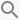# ANOVA Explained: How to compare differences of meansWhy do we use analysis of variance (ANOVA) when we’re interested in the differences among means? And how do ANOVA tests work? We’ll try and lay it out simply for you.

ANOVA is used to compare differences of means among more than two groups. It does this by looking at variation in the data and where that variation is found (hence its name). Specifically, ANOVA compares the amount of variation between groups with the amount of variation within groups. It can be used for both observational and experimental studies.

When we take samples from a population, we expect each sample mean to differ simply because we are taking a sample rather than measuring the whole population; this is called sampling error but is often referred to more informally as the effects of “chance”. Thus, we always expect there to be some differences in means among different groups.

The question is: is the difference among groups greater than that expected to be caused by chance? In other words, is there likely to be a true (real) difference in the population mean?

Although it may seem difficult at first, statistics becomes much easier if you understand what the test is doing rather than blindly applying it. This isn’t a “dummies”-level explanation, but hopefully ANOVA will become clear by following the steps below.

## The ANOVA model

Mathematically, ANOVA can be written as:

x ij = μ i + ε ij

where x are the individual data points (i and j denote the group and the individual observation), ε  is the unexplained variation and the parameters of the model (μ) are the population means of each group. Thus, each data point (xij) is its group mean plus error.

## Hypothesis testing

Like other classical statistical tests, we use ANOVA to calculate a test statistic (the F-ratio) with which we can obtain the probability (the P-value) of obtaining the data assuming the null hypothesis. A significant P-value (usually taken as P<0.05) suggests that at least one group mean is significantly different from the others.

Null hypothesis: all population means are equal

Alternative hypothesis: at least one population mean is different from the rest.

## Calculation of the F ratio

ANOVA separates the variation in the dataset into 2 parts: between-group and within-group. These variations are called the sums of squares, which can be seen in the equations below.

### Step 1) Variation between groups (Fig 1)

The between-group variation (or between-group sums of squares, SS) is calculated by comparing the mean of each group with the overall mean of the data.

Specifically, this is: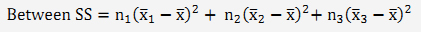i.e., by adding up the square of the differences between each group mean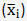and the overall population mean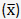, multiplied by sample size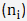, assuming we are comparing three groups (i = 1, 2 or 3).

We then divide the BSS by the number of degrees of freedom (this is like sample size, except it is n-1, because the deviations must sum to zero, and once you know n-1, the last one is also known) to get our estimate of the mean variation between groups.

### Step 2) Variation within groups  (Fig 1)

The within-group variation (or the within-group sums of squares) is the variation of each observation from its group mean.

SSR = s2group1 (ngroup1 – 1) + s2group2 (ngroup2 – 1) + s2group3 (ngroup3 – 1)

i.e., by adding up the variance of each group times by the degrees of freedom of each group. Note, you might also come across the total SS (sum of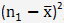). Within SS is then Total SS minus Between SS.

As before, we then divide by the total degrees of freedom to get the mean variation within groups.

### Step 3) The F ratio

The F ratio is then calculated as: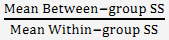If the average difference between groups is similar to that within groups, the F ratio is about 1. As the average difference between groups becomes greater than that within groups, the F ratio becomes larger than 1.

To obtain a P-value, it can be tested against the F-distribution of a random variable with the degrees of freedom associated with the numerator and denominator of the ratio. The P-value is the probably of getting that F ratio or a greater one. Larger F-ratios gives smaller P-values.

## Assumptions of ANOVA

• The response is normally distributed
• Variance is similar within different groups
• The data points are independent

Example: A researcher conducted a study to investigate the effect of teaching method on the reading ability of schoolchildren. Fifteen children were randomly allocated to one of three teaching methods and their reading ability measured on a validated score. The ANOVA table below shows that the F-ratio is 52.022, which gives a p-value of P<0.001 (with degrees of freedom 2 and 42). Figure 1 present the data, showing the between-group variation and within-group variation.

Analysis of Variance (ANOVA) Table

Response: data

 Df SS Mean SS F ratio P(>F) Between-group 2 44.475 22.2373 52.022 4.306e-12*** Within-group 42 17.953 0.4275

The average amount of variation between groups is greater than that within groups (see graph below), which means that the F-ratio is large and the P-value is very small.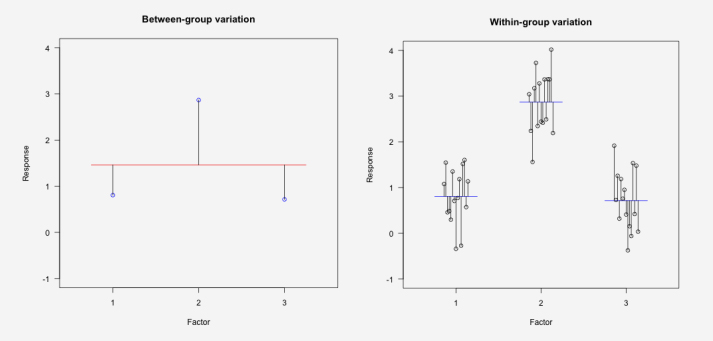Fig. 1 Data from a study comparing the reading scores of children (response) according to teaching method (a factor with 3 levels) (left) – the amount of variation between groups and (right) – the amount of variation within groups. ANOVA tests whether the average amount of variation between groups is greater than the average amount of variation within groups. Black lines show the deviations (i.e., the variation). In the left graph, the red line is the overall mean of the data while the blue points are the group means. In the right graph, the blue lines show the group means and black circles show the data points.

## ANOVA and…

### The t-test

t-test is used when comparing two groups while ANOVA is used for comparing more than 2 groups. In fact, if you calculate the p-value using ANOVA for 2 groups, you will get the same results as the t-test.

### Linear regression

Linear regression is used to analyze continuous relationships; however, regression is essentially the same as ANOVA. In ANOVA, we calculate means and deviations of our data from the means. In linear regression, we calculate the best line through the data and calculate the deviations of the data from this line. The F ratio can be calculated in both.

To be sure your use of ANOVA, statistics , and effective scientific language are in order, scientific review and expert editing are worth many times their price. They can help you avoid major revisions (or rejection) and get published sooner. Explore your options here. We want to see you get published.